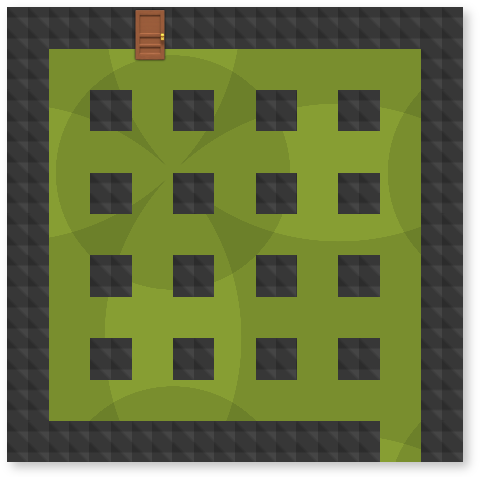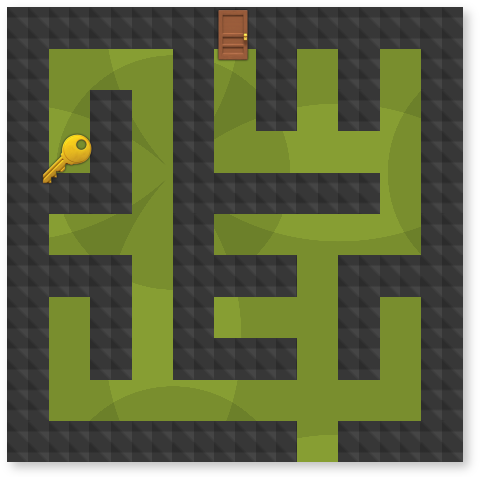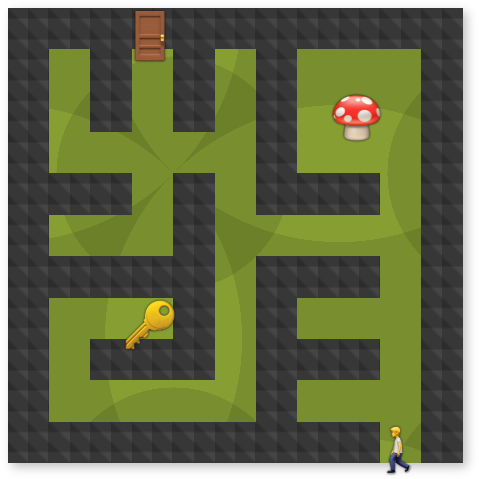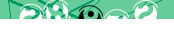# JavaScript: Random Maze Generator

Following many requests we've ported our PHP maze generating class to JavaScript and are making it publicly available.

This is a basic version which will create a maze of the specified dimensions, with an entrance, exit, and a cunningly placed key to be retrieved en route. You can find a more advanced version linked below.

## Working Demonstration

Below you should see a randomly generated maze with 16 columns and 12 rows. This maze is not interactive, but can be made so by subsequently applying our Maze Game code presented earlier. There are also no monsters or treasure.

Unlike our earlier examples which used PHP, this maze is generated entirely in your browser, so you can (re-)generate mazes to your heart's content:

Maze Generator

## MazeBuilder Source Code

``` // Original JavaScript code by Chirp Internet: chirpinternet.eu // Please acknowledge use of this code by including this header. class MazeBuilder {   constructor(width, height) {     this.width = width;     this.height = height;     this.cols = 2 * this.width + 1;     this.rows = 2 * this.height + 1;     this.maze = this.initArray([]);     // place initial walls     this.maze.forEach((row, r) => {       row.forEach((cell, c) => {         switch(r)         {           case 0:           case this.rows - 1:             this.maze[r][c] = ["wall"];             break;           default:             if((r % 2) == 1) {               if((c == 0) || (c == this.cols - 1)) {                 this.maze[r][c] = ["wall"];               }             } else if(c % 2 == 0) {               this.maze[r][c] = ["wall"];             }         }       });       if(r == 0) {         // place exit in top row         let doorPos = this.posToSpace(this.rand(1, this.width));         this.maze[r][doorPos] = ["door", "exit"];       }       if(r == this.rows - 1) {         // place entrance in bottom row         let doorPos = this.posToSpace(this.rand(1, this.width));         this.maze[r][doorPos] = ["door", "entrance"];       }     });     // start partitioning     this.partition(1, this.height - 1, 1, this.width - 1);   }   initArray(value) {     return new Array(this.rows).fill().map(() => new Array(this.cols).fill(value));   }   rand(min, max) {     return min + Math.floor(Math.random() * (1 + max - min));   }   posToSpace(x) {     return 2 * (x-1) + 1;   }   posToWall(x) {     return 2 * x;   }   inBounds(r, c) {     if((typeof this.maze[r] == "undefined") || (typeof this.maze[r][c] == "undefined")) {       return false; // out of bounds     }     return true;   }   shuffle(array) {     // sauce: https://stackoverflow.com/a/12646864     for(let i = array.length - 1; i > 0; i--) {       const j = Math.floor(Math.random() * (i + 1));       [array[i], array[j]] = [array[j], array[i]];     }     return array;   }   partition(r1, r2, c1, c2) {     // create partition walls     // ref: https://en.wikipedia.org/wiki/Maze_generation_algorithm#Recursive_division_method     let horiz, vert, x, y, start, end;     if((r2 < r1) || (c2 < c1)) {       return false;     }     if(r1 == r2) {       horiz = r1;     } else {       x = r1+1;       y = r2-1;       start = Math.round(x + (y-x) / 4);       end = Math.round(x + 3*(y-x) / 4);       horiz = this.rand(start, end);     }     if(c1 == c2) {       vert = c1;     } else {       x = c1 + 1;       y = c2 - 1;       start = Math.round(x + (y - x) / 3);       end = Math.round(x + 2 * (y - x) / 3);       vert = this.rand(start, end);     }     for(let i = this.posToWall(r1)-1; i <= this.posToWall(r2)+1; i++) {       for(let j = this.posToWall(c1)-1; j <= this.posToWall(c2)+1; j++) {         if((i == this.posToWall(horiz)) || (j == this.posToWall(vert))) {           this.maze[i][j] = ["wall"];         }       }     }     let gaps = this.shuffle([true, true, true, false]);     // create gaps in partition walls     if(gaps) {       let gapPosition = this.rand(c1, vert);       this.maze[this.posToWall(horiz)][this.posToSpace(gapPosition)] = [];     }     if(gaps) {       let gapPosition = this.rand(vert+1, c2+1);       this.maze[this.posToWall(horiz)][this.posToSpace(gapPosition)] = [];     }     if(gaps) {       let gapPosition = this.rand(r1, horiz);       this.maze[this.posToSpace(gapPosition)][this.posToWall(vert)] = [];     }     if(gaps) {       let gapPosition = this.rand(horiz+1, r2+1);       this.maze[this.posToSpace(gapPosition)][this.posToWall(vert)] = [];     }     // recursively partition newly created chambers     this.partition(r1, horiz-1, c1, vert-1);     this.partition(horiz+1, r2, c1, vert-1);     this.partition(r1, horiz-1, vert+1, c2);     this.partition(horiz+1, r2, vert+1, c2);   }   isGap(...cells) {     return cells.every((array) => {       let row, col;       [row, col] = array;       if(this.maze[row][col].length > 0) {         if(!this.maze[row][col].includes("door")) {           return false;         }       }       return true;     });   }   countSteps(array, r, c, val, stop) {     if(!this.inBounds(r, c)) {       return false; // out of bounds     }     if(array[r][c] <= val) {       return false; // shorter route already mapped     }     if(!this.isGap([r, c])) {       return false; // not traversable     }     array[r][c] = val;     if(this.maze[r][c].includes(stop)) {       return true; // reached destination     }     this.countSteps(array, r-1, c, val+1, stop);     this.countSteps(array, r, c+1, val+1, stop);     this.countSteps(array, r+1, c, val+1, stop);     this.countSteps(array, r, c-1, val+1, stop);   }   getKeyLocation() {     let fromEntrance = this.initArray();     let fromExit = this.initArray();     this.totalSteps = -1;     for(let j = 1; j < this.cols-1; j++) {       if(this.maze[this.rows-1][j].includes("entrance")) {         this.countSteps(fromEntrance, this.rows-1, j, 0, "exit");       }       if(this.maze[j].includes("exit")) {         this.countSteps(fromExit, 0, j, 0, "entrance");       }     }     let fc = -1, fr = -1;     this.maze.forEach((row, r) => {       row.forEach((cell, c) => {         if(typeof fromEntrance[r][c] == "undefined") {           return;         }         let stepCount = fromEntrance[r][c] + fromExit[r][c];         if(stepCount > this.totalSteps) {           fr = r;           fc = c;           this.totalSteps = stepCount;         }       });     });     return [fr, fc];   }   placeKey() {     let fr, fc;     [fr, fc] = this.getKeyLocation();     this.maze[fr][fc] = ["key"];   }   display(id) {     this.parentDiv = document.getElementById(id);     if(!this.parentDiv) {       alert("Cannot initialise maze - no element found with id \"" + id + "\"");       return false;     }     while(this.parentDiv.firstChild) {       this.parentDiv.removeChild(this.parentDiv.firstChild);     }     const container = document.createElement("div");     container.id = "maze";     container.dataset.steps = this.totalSteps;     this.maze.forEach((row) => {       let rowDiv = document.createElement("div");       row.forEach((cell) => {         let cellDiv = document.createElement("div");         if(cell) {           cellDiv.className = cell.join(" ");         }         rowDiv.appendChild(cellDiv);       });       container.appendChild(rowDiv);     });     this.parentDiv.appendChild(container);     return true;   } } ```

expand code box

Please note that we've written the MazeBuilder class using ES6 class notation, meaning that it may not work in some older browsers.

## How does it work?

The constructor() creates an empty matrix which is then walled (Figure 1) and partitioned (Figure 2) according to the recursive division algorithm which you can find details of under References below.Placing the key with placeKey() is the most complicated part of the code as we first traverse the maze from both the start point and the end point with countSteps() and use that to calculate where the key should go to be as far away as possible from both those points (Figure 3).

Finally, the display() method generates and inserts the maze into the page using HTML with classes to indicate the position of walls and other elements.You may notice that the isGap() method is way more complicated than necessary for the purpose used, but the reason for that will become evident in the more advanced version where we start finessing the maze layout. Similarly, the totalSteps value is only used when the maze becomes interactive (Figure 4).

## Code for generating a maze

All you need now in order to start generating your own mazes is the following code, including a CSS file and the above JavaScript file:

```<link rel="stylesheet" type="text/css" href="/mazing.css"> <div id="maze_container"><!-- --></div> <script src="/maze-builder.js"></script> <script> let Maze = new MazeBuilder(16, 12); Maze.placeKey(); Maze.display("maze_container"); </script>```

You will need to copy these files to your own server and include them from there to avoid our hot-linking protection.

## References

< JavaScript

#### Send a message to The Art of Web: